Jarvis' Blog (总有美丽的风景让人流连) 总有美丽的风景让人流连

# 注意力机制和 Transformer (Attention and Transformer)

2022-03-29
Jarvis
Post

Self-Attention 和 Transformer 自从问世就成为了自然语言处理领域的新星. 得益于全局的注意力机制和并行化的训练, 基于 Transformer 的自然语言模型能够方便的编码的长距离依赖关系, 同时在大规模自然语言数据集上并行训练成为可能.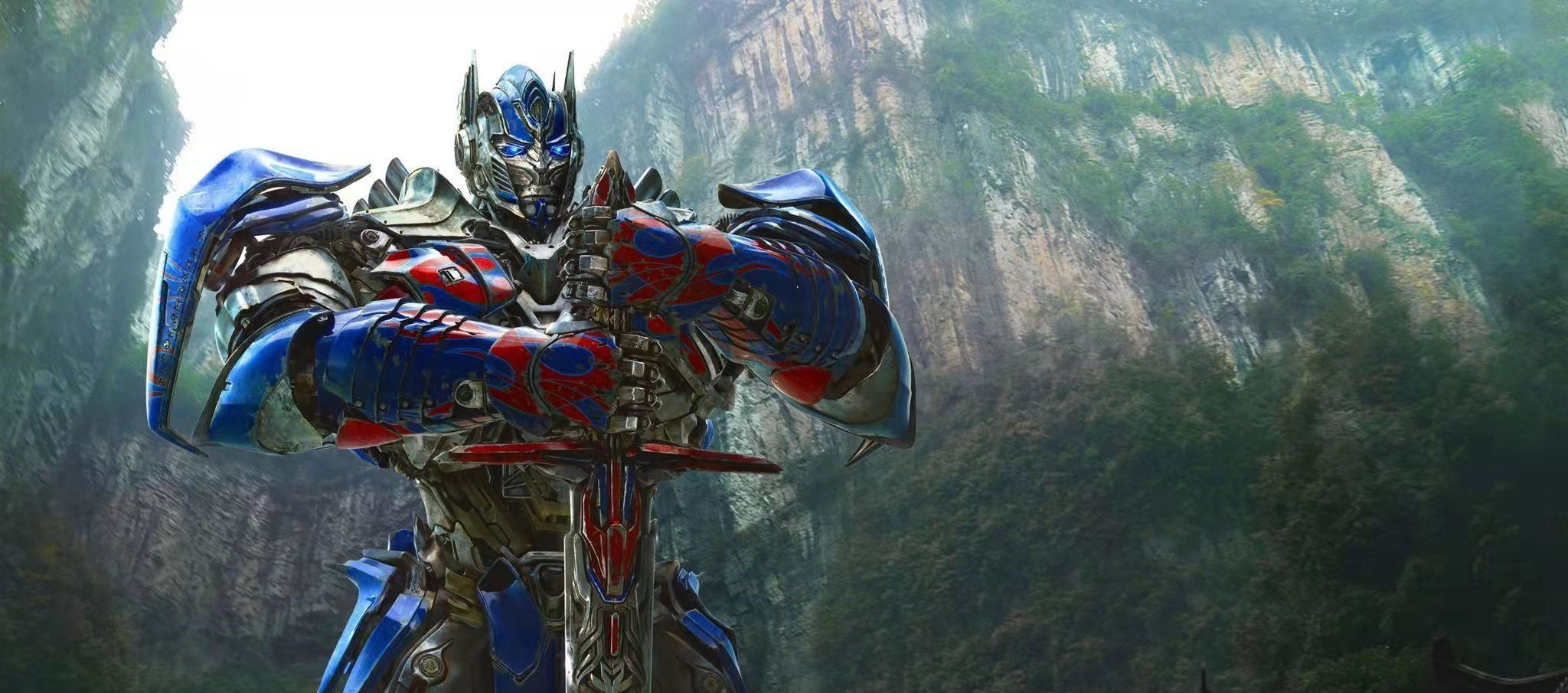## 1. 基本概念

Self-Attention 和 Transformer1 自从问世就成为了自然语言处理 (Natural Language Processing, NLP) 领域的新星. 得益于全局的注意力机制和并行化的训练, 基于 Transformer 的自然语言模型能够方便的编码的长距离依赖关系, 同时在大规模自然语言数据集上并行训练成为可能.

### 1.1 Self-Attention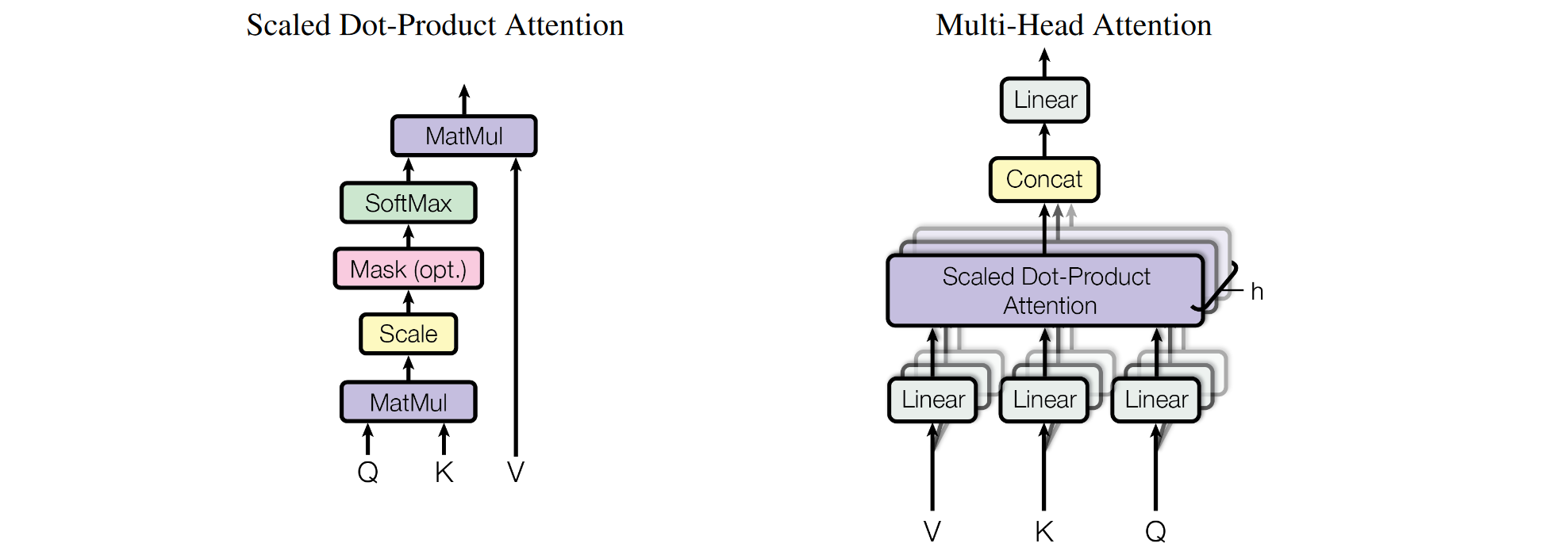Self-Attention 在空间维度做全局吸收. 假设输入特征 $$F\in\mathbb{R}^{b\times l\times d_0}$$, 其中 $$b$$ 是批大小 (batch size), $$l$$ 是空间维度 (NLP 中是序列长度, CV 中是图像长宽的乘积 $$h\times w$$), $$d_0$$ 是特征维度.

1. 首先对 $$F$$ 做线性变化得到 $$Q=FW_Q, K=FW_K, V=FW_V \in\mathbb{R}^{b\times l\times d}$$
2. 计算 $$Q, K$$ 的相似度矩阵 $$S = QK^T \in\mathbb{R}^{b\times l\times l}$$
3. 缩放相似度矩阵 $$S / \sqrt{d}$$
4. Softmax 沿 $$K$$ 的轴归一化得到权重 $$W=\text{Softmax}(S / \sqrt{d})$$
5. 对 $$V$$ 加权求和得到新的特征
$\text{Attention}(Q, K, V) = \text{Softmax}(\frac{QK^T}{\sqrt{d}})V$

1. Self-Attention 的 $$Q, K, V$$ 来自同一个输入特征 $$F$$. 由于矩阵化计算, 输入序列(图像)的空间点是并行处理的, 因此模型无法捕获序列的顺序信息和图像的空间位置信息. 所以需要对 $$Q, K, V$$ 不同的空间点加上不同的位置编码 (positional encoding). 为了清晰, 上面的公式略去了位置编码.
2. Cross-Attention 的 $$Q$$ 来自输入特征 $$F_1$$, 而 $$K, V$$ 来自输入特征 $$F_2$$
3. 缩放. 当 $$C_1$$ 较大时, 相似度矩阵在 Softmax 后梯度会偏小, 导致学习困难. 见图, Softmax 的梯度在 $$x$$ 绝对值增大时梯度迅速衰减.
4. 加权. 根据当前点与其他点的相似度, 使用 $$V$$ 重构当前点的信息.
5. 加入残差后 $$F_{out} = F_{in} + \text{Attention}(F_{in})$$, 那么注意力学习的就是输出和输出的变化:
• $$\text{Self-Attention}(F_{in}) = F_{out} - F_{in}$$, 这可以看作输入特征吸收了自己其他位置的信息
• $$\text{Cross-Attention}(F_{in}^{(q)}, F_{in}^{(k)}) = F_{out}^{(q)} - F_{in}^{(q)}$$, 这可以看作输入特征 $$F_{in_q}$$ 吸收了 $$F_{in_k}$$ 的信息.

### 1.2 Transformer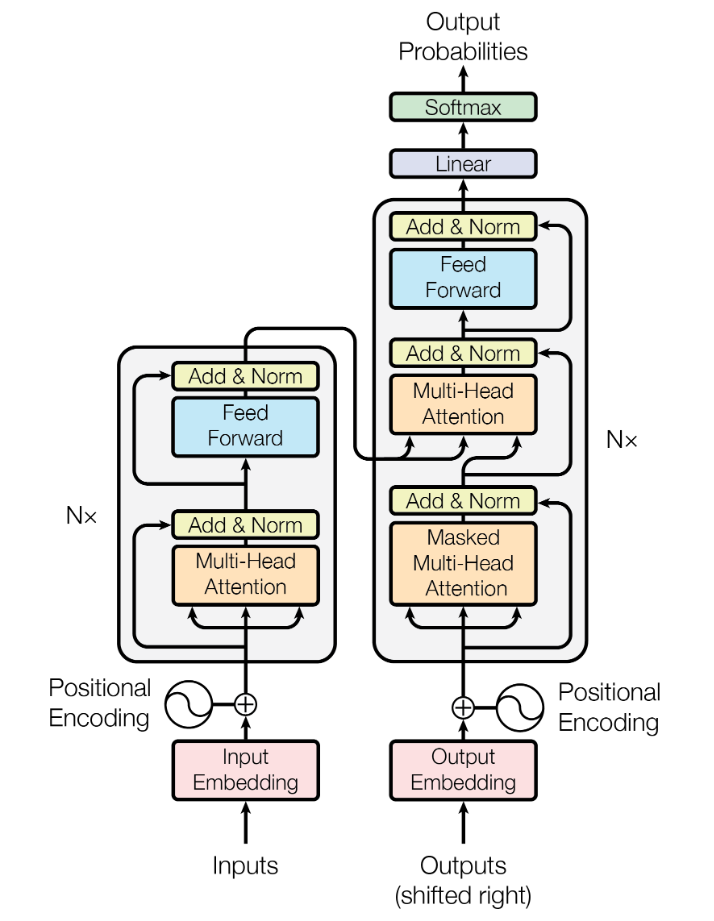## 2. Vision-Transformer (ViT)

### 2.1 模型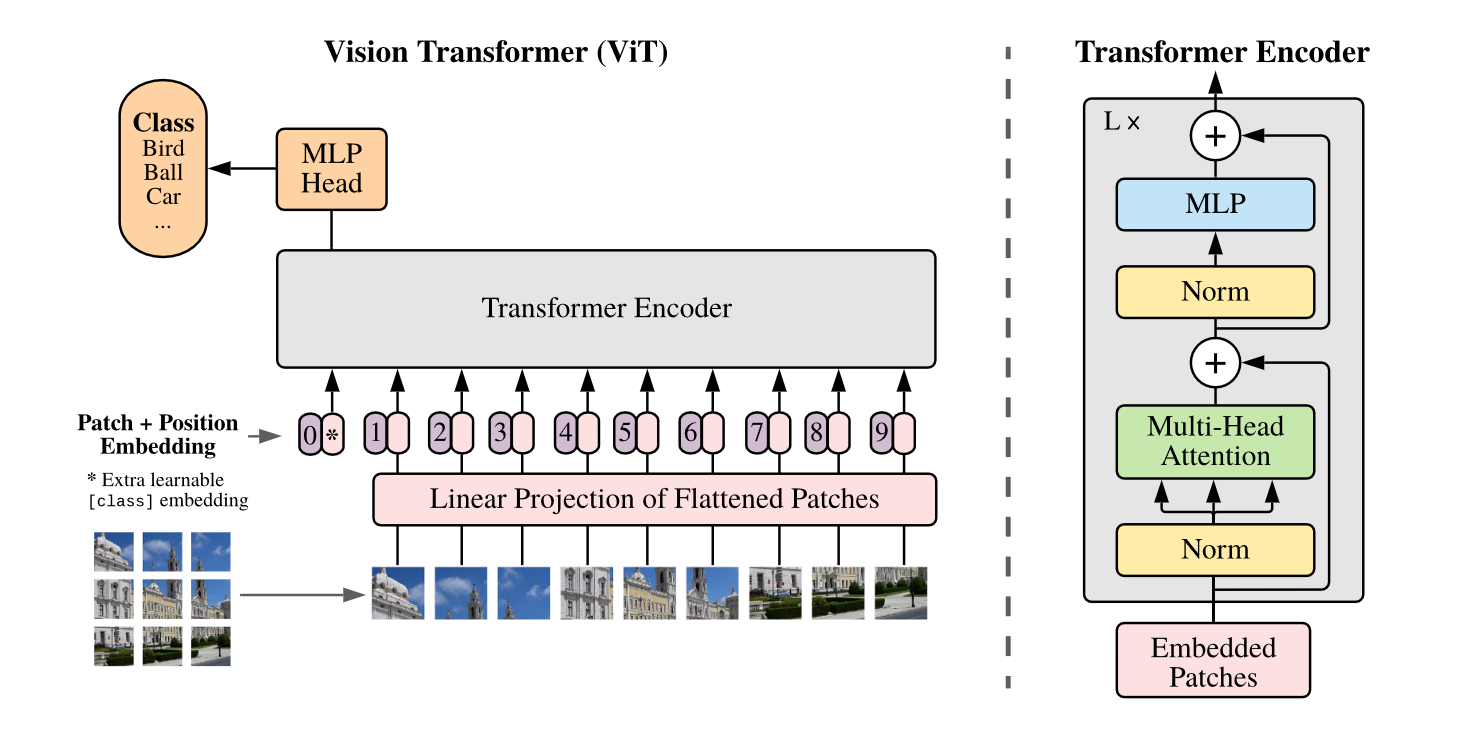ViT2 创造性地把图像分类问题看成序列分类问题.

ViT 不需要解码器.

ViT 可以用公式表示为:

\begin{align} \mathbf{z}_0 &= [\mathbf{x}_{\text{class}}; \mathbf{x}_p^1\mathbf{E}; \mathbf{x}_p^2\mathbf{E}; \cdots; \mathbf{x}_p^N\mathbf{E};] + \mathbf{E}_{\text{pos}}, & \mathbf{E}&\in\mathbb{R}^{(P^2\cdot C)\times D}, \mathbf{E}_{\text{pos}}\in\mathbb{R}^{(N + 1)\times D}, \\ \mathbf{z}'_l &= \text{MSA}(\text{LN}(\mathbf{z}_{l-1})) + \mathbf{z}_{l-1}, & l &=1\dots L, \\ \mathbf{z}_l &= \text{FFN}(\text{LN}(\mathbf{z}'_l)) + \mathbf{z}'_l, & l &=1\dots L, \\ \mathbf{y} &= \text{LN}(\mathbf{z}_L^0) \end{align}

### 2.2 数据集

ImageNet 1k 1.3M avg 469 x 387
ImageNet 21k 14M avg 469 x 387
ImageNet Real 21k 14M avg 469 x 387
JFT 18k 303M -
• ImageNet Real3 是对 ImageNet 的标签进行修正后的数据集

### 2.3 结构和参数

ViT-Small 12 384 1536 12 22M
ViT-Base 12 768 3072 12 86M
ViT-Large 24 1024 4096 16 307M
ViT-Huge 32 1280 5120 16 632M

#### 参数量计算

ViT 的参数依次包含在以下层:

Input Embedding Layer (input dim $$P^2\cdot C$$, embedding dim $$D$$) $$P^2CD + D$$
Class Token $$D$$
Positional Embedding (number patches $$N$$) $$(N + 1)D$$
Attention (layers $$L$$, heads $$H$$) $$L(4D^2 + 4D)$$
MLP (MLP hidden dim $$D'=4D$$) $$L(2DD' + D' + D)$$
Layer Norm $$L\cdot4D + 2D$$

$\text{#Transformer} = (P^2C + N + 5)D + L(12D^2 + 13D)$

### 2.4 结果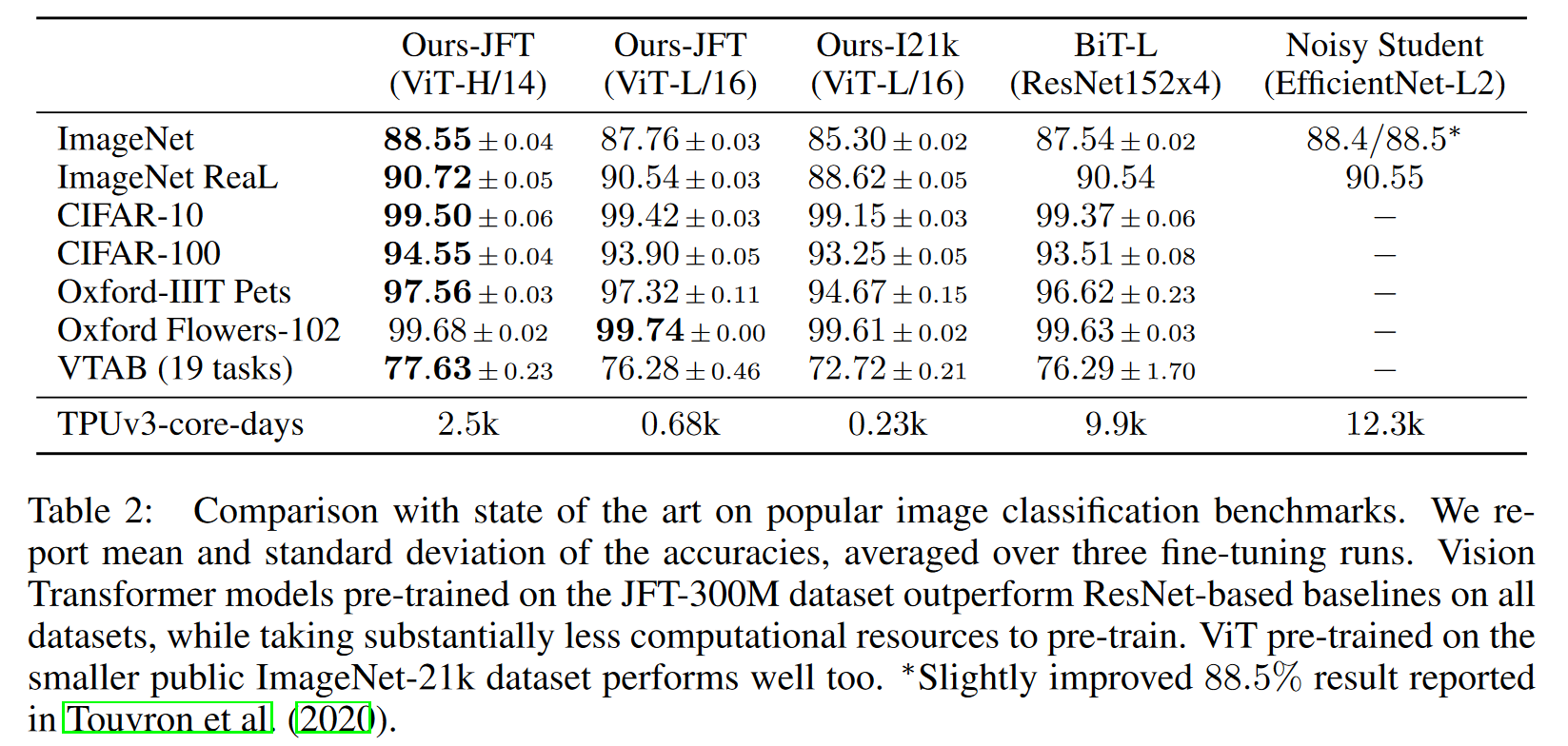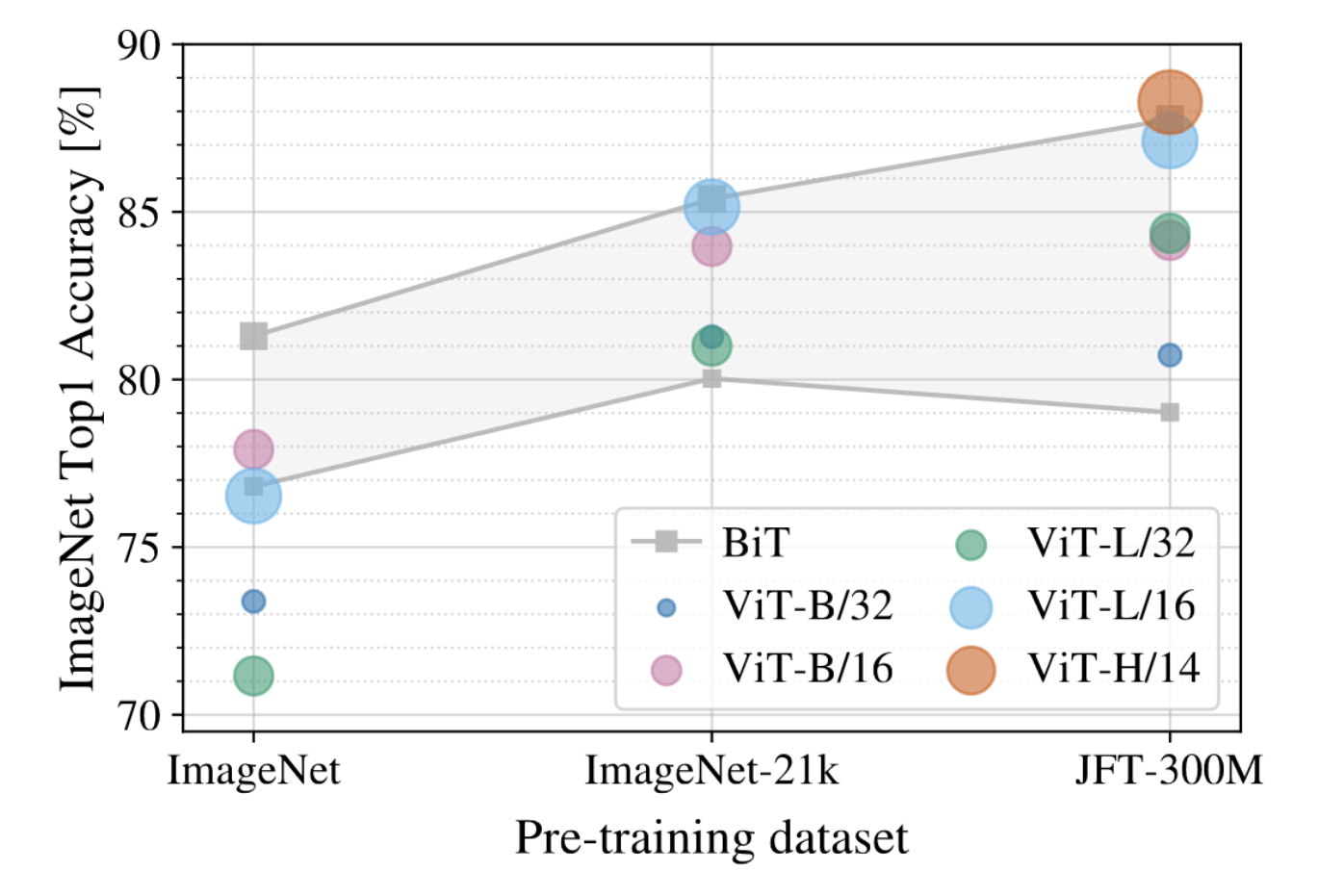• 输入的图像序列也可以替换为卷积网络的特征图. 小模型使用特征图更好一些, 大模型两种输入序列的差距基本消失.
• ViT 可以达到和卷积网络相似的性能, 同时训练速度更快.
• ViT 在更大规模的数据集上 (JFT) 上的表现最好, 在稍小规模的数据集上 (ImageNet) 表现不如卷积网络.

## 3. Data-efficient image Transformer (DeiT)

### 3.1 模型

ViT 需要使用大量的数据 (如 JFT) 先预训练, 然后在 ImageNet 上微调才能达到和卷积网络相同的性能.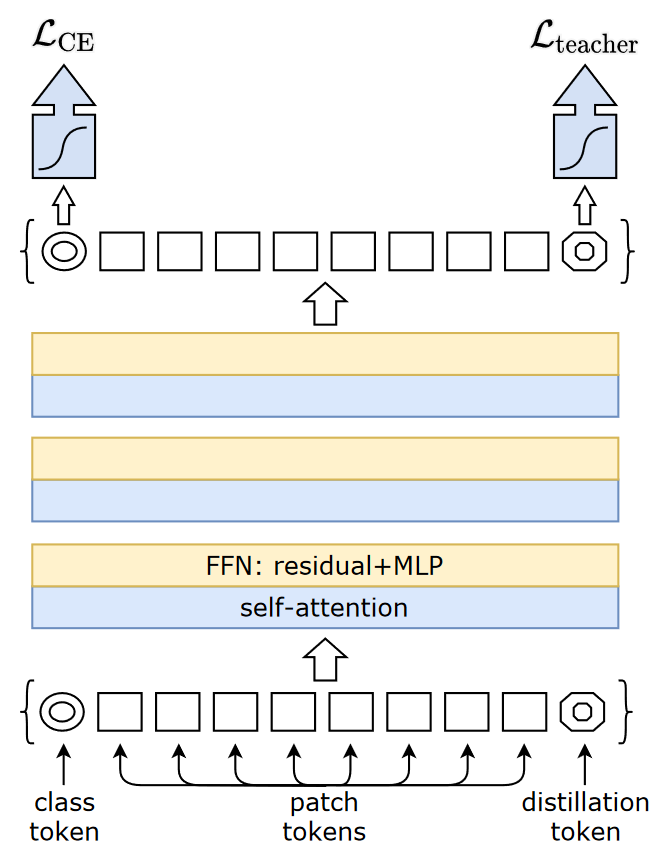DeiT 引入蒸馏来解决该问题. 如上图, DeiT 额外增加一个 distillation token, 并用该 token 对应的输出计算蒸馏损失. 还提出了 Hard Distillation 的方法. 即使用 teacher 的预测结果作为标签, 直接使用交叉熵训练 (不使用 KL divergence):

$\mathcal{L}^{\text{hardDistill}} = \frac12\mathcal{L}_{\text{CE}}(\phi(Z_s), y) + \frac12\mathcal{L}_{\text{CE}}(\phi(Z_s), y_t).$

### 3.2 结构

DeiT-Tiny 12 192 3 5M
DeiT-Small 12 384 6 22M
DeiT-Base 12 768 12 86M

### 3.3 结果

DeiT 的主要结果如下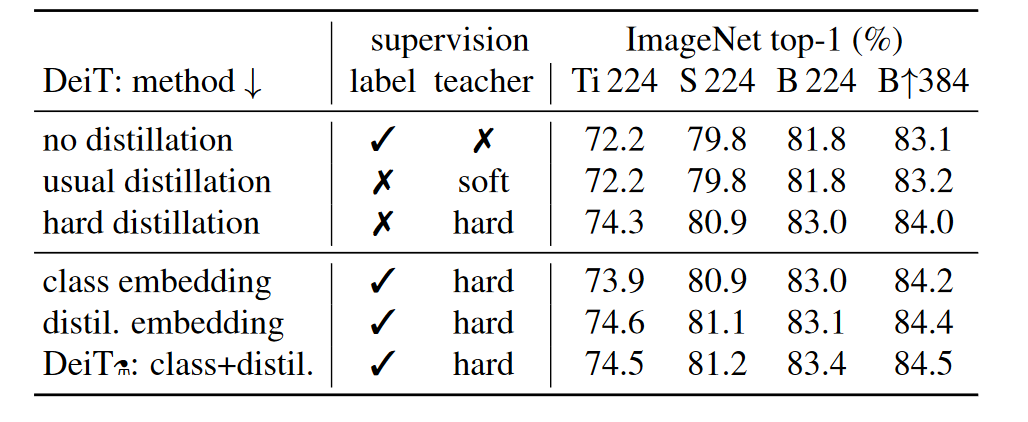• 下三行是使用了额外的 distillation token 的结果. 使用该 token 的效果更好.
• 使用末尾 distillation embedding 做分类器的效果要好于开头 class embedding 做分类器.
• DeiT 分析了不同蒸馏标签的影响, 发现用 hard label 更好.
• 使用 Convnet teacher 要好于使用 Transformer teacher. (见论文表2)
1. Attention is all you need
Ashish Vaswani, Noam Shazeer, Niki Parmar, Jakob Uszkoreit, Llion Jones, Aidan N. Gomez, Lukasz Kaiser, Illia Polosukhin
[html] in NeruIPS 2017.

2. An Image is Worth 16x16 Words: Transformers for Image Recognition at Scale
Alexey Dosovitskiy, Lucas Beyer, Alexander Kolesnikov, et al.
[html] in ICLR 2021.

3. Are we done with ImageNet?
Lucas Beyer, Olivier J. Hénaff, Alexander Kolesnikov, Xiaohua Zhai, Aäron van den Oord
[html] in arXiv 2006.

Content